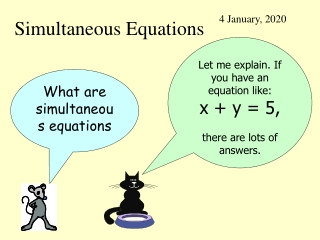DownloadDownload PresentationSimultaneous Equations

# Simultaneous Equations

Télécharger la présentation## Simultaneous Equations

- - - - - - - - - - - - - - - - - - - - - - - - - - - E N D - - - - - - - - - - - - - - - - - - - - - - - - - - -
##### Presentation Transcript

1. 4 January, 2020 Simultaneous Equations Let me explain. If you have an equation like: x + y = 5, there are lots of answers. What are simultaneous equations

2. Here are some of these answers I can think of some more because 1.5 + 3.5 = 5 so x = 1.5 and y = 3.5 etc.

3. There are lots of answers that fit the equation x + y = 5 That’s right but suppose that we have another equation to go with x + y = 5 and the x and y must be the same numbers for both equations. x + y = 5 x – y = 1 Like this

4. x + y = 5 x – y = 1 The only values that will fit both equations are x = 3 and y = 2. Equations like this are called simultaneous equations. 3 + 2 = 5 3 – 2 = 1

5. Here is a method for solving simultaneous equations x +y=9 x –y= 5 • Make sure that the middles are the same • y • y

6. Here is a method for solving simultaneous equations x +y=9 x –y= 5 • Make sure that the middles are the same • If the signs are different ADD • (+ y) and (– y) have different signs so ADD

7. Here is a method for solving simultaneous equations x +y=9 x –y= 5 2x= 14 • Make sure that the middles are the same • If the signs are different ADD • x+x=2x and (+ y ) + (- y ) = 0 • and 9 + 5 = 14

8. Here is a method for solving simultaneous equations x +y=9 x –y= 5 2x= 14 x =7 • Make sure that the middles are the same • If the signs are different ADD • Find the value of x • 2 x = 14 • x = 14 ÷ 2 • x = 7

9. Here is a method for solving simultaneous equations x +y=9 x –y= 5 2x= 14 x =7 x +y=9 7+y=9 y = 9 – 7 y =2 • Make sure that the middles are the same • If the signs are different ADD • Find the value of x • Use this to find the value ofy • 7 + y = 9 • y = 9 – 7 • y = 2

10. Here is another pair of simultaneous equations 2x +y=11 x –y= 4 To solve, follow the steps

11. 2x +y=11 x –y= 4 3x= 15 • Make sure that the middles are the same • If the signs are different ADD • 2x + x = 3x • (+y) + (–y) = 0 • 11 + 4 = 15

12. 2x +y=11 x –y= 4 3x= 15 x =5 • Make sure that the middles are the same • If the signs are different ADD • Find the value of x • 3x= 15 • x =15 ÷ 3 • x = 5

13. 2x +y=11 x –y= 4 3x= 15 x =5 2x + y= 11 10 +y= 11 • Make sure that the middles are the same • If the signs are different ADD • Find the value of x • Use this to find the value ofy

14. 2x +y=11 x –y= 4 3x = 15 x =5 2x + y= 11 10 +y= 11 y = 11 – 10 y = 1 • Make sure that the middles are the same • If the signs are different ADD • Find the value of x • Use this to find the value ofy

15. When the middle signs are the same 2x + y = 14 x + y = 4 The same

16. 2x +y=14 x +y= 9 x= 5 2x + y= 14 10 +y= 14 y = 14 – 10 y = 4 • Make sure that the middles are the same • If the signs are the same SUBTRACT • Find the value of x • Use this to find the value ofy

17. Make sure that the middles are the same • If the signs are the Same SUBTRACT • If the signs are Different ADD • 3. Find the value of x • 4. Use this to find the value ofy